Maths-
General
Easy

Question

# Arrange the numbers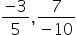and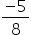in ascending orderHint:

## The correct answer is: ascending order

### Given rational numbers areand.Express the rational numbers with positive denominator by multiplying with -1.Here,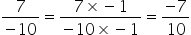So, Now we have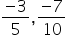and.By taking LCM of denominators 5,10 and 8, we get LCM as 40On multiplying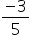with 8, we get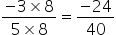On multiplying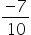with 4, we get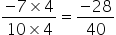On multiplyingwith 5, we get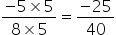On comparing the above fractions with same denominator,we have  -28 < -25 < -24Hence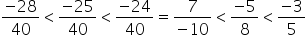So ascending order is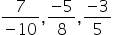.#### With Turito Foundation.#### Get an Expert Advice From Turito.